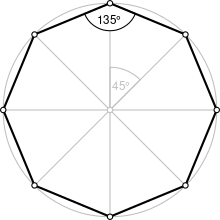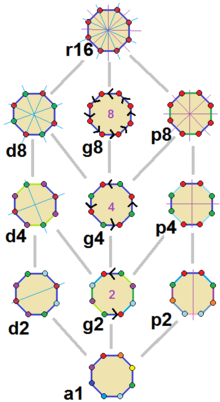# ثماني الأضلاع

في الهندسة الرياضية، ثماني الأضلاع هو مضلع له 8 أضلاع.

Regular octagonA regular octagon
النوعمضلع منتظم
الأضلاع والرؤوس{{{p8 جانب}}}
رمز شلفلي{{{p8-شلفلي}}}
مخططات كوكستر-دنكنمجموعة التماثلDihedral (D8), order 2×8
الزاوية الداخلية (الدرجات)135°
الخصائصConvex, cyclic, equilateral, isogonal, isotoxal

A regular octagon has Schläfli symbol {8}  and can also be constructed as a quasiregular truncated square, t{4}, which alternates two types of edges. A truncated octagon, t{8} is a hexadecagon, {16}. A 3D analog of the octagon can be the rhombicuboctahedron with the triangular faces on it like the replaced edges, if one considers the octagon to be a truncated square.Note: We can make Octagon by compass & by protector. There are 2 ways of making an Octagon. They are; 1^st= On the basis of side & 2^nd= On the basis of radious(Circle)

. . . . . . . . . . . . . . . . . . . . . . . . . . . . . . . . . . . . . . . . . . . . . . . . . . . . . . . . . . . . . . . . . . . . . . . . . . . . . . . . . . . . . . . . . . . . . . . . . . . . . . . . . . . . . . . . . . . . . . . . . . . . . . . . . . . . . . . . . . . . . . . . . . . . . . . . . . . . . . . . . . . . . . . .

## خصائص المثمّن العام

The sum of all the internal angles of any octagon is 1080°. As with all polygons, the external angles total 360°.

If squares are constructed all internally or all externally on the sides of an octagon, then the midpoints of the segments connecting the centers of opposite squares form a quadrilateral that is both equidiagonal and orthodiagonal (that is, whose diagonals are equal in length and at right angles to each other).:Prop. 9

The midpoint octagon of a reference octagon has its eight vertices at the midpoints of the sides of the reference octagon. If squares are constructed all internally or all externally on the sides of the midpoint octagon, then the midpoints of the segments connecting the centers of opposite squares themselves form the vertices of a square.:Prop. 10

## ثماني منتظم (مثمن)

• المثمن هو ثماني أضلاع أضلاعه متساوية وزواياه متساوية.
• قياس الزاوية الداخلية يساوي 135°.
• مجموع قياسات زواياه الداخلية 1080°.
• مساحة المثمن المنتظم الذي طول ضلعه a تعطى بالعلاقة:
$A=2\cot {\frac {\pi }{8}}a^{2}=2(1+{\sqrt {2}})a^{2}\simeq 4.828427\,a^{2}.$

وتعطى المساحة بدلالة R نصف قطر الدائرة المحيطة به بالعلاقة:

$A=4\sin {\frac {\pi }{4}}R^{2}=2{\sqrt {2}}R^{2}\simeq 2.828427\,R^{2}.$

وتعطى المساحة بدلالة r نصف قطر الدائرة المحاطة داخله بالعلاقة:

$A=8\tan {\frac {\pi }{8}}r^{2}=8({\sqrt {2}}-1)r^{2}\simeq 3.3137085\,r^{2}.$

### الأقطار

The regular octagon, in terms of the side length a, has three different types of diagonals:

• القطر القصير;
• Medium diagonal (also called span or height), which is twice the length of the inradius;
• Long diagonal, which is twice the length of the circumradius.

The formula for each of them follows from the basic principles of geometry. Here are the formulas for their length:[بحاجة لمصدر]

• القطر القصير: $a{\sqrt {2+{\sqrt {2}}}}$  ;
• Medium diagonal: $(1+{\sqrt {2}})a$  ; (silver ratio times a)
• Long diagonal: $a{\sqrt {4+2{\sqrt {2}}}}$  .

### الإنشاء والخصائص الابتدائية

A regular octagon at a given circumcircle may be constructed as follows:

1. Draw a circle and a diameter AOE, where O is the center and A, E are points on the circumcircle.
2. Draw another diameter GOC, perpendicular to AOE.
3. (Note in passing that A,C,E,G are vertices of a square).
4. Draw the bisectors of the right angles GOA and EOG, making two more diameters HOD and FOB.
5. A,B,C,D,E,F,G,H are the vertices of the octagon.

A regular octagon can be constructed using a straightedge and a compass, as 8 = 23, a power of two:

The regular octagon can be constructed with meccano bars. Twelve bars of size 4, three bars of size 5 and two bars of size 6 are required.

Each side of a regular octagon subtends half a right angle at the centre of the circle which connects its vertices. Its area can thus be computed as the sum of 8 isosceles triangles, leading to the result:

${\text{Area}}=2a^{2}({\sqrt {2}}+1)$

for an octagon of side a.

### الإحداثيات القياسية

The coordinates for the vertices of a regular octagon centered at the origin and with side length 2 are:

• (±1, ±(1+2))
• (±(1+2), ±1).

### تشريح

Coxeter states that every zonogon (a 2m-gon whose opposite sides are parallel and of equal length) can be dissected into m(m-1)/2 parallelograms. In particular this is true for regular polygons with evenly many sides, in which case the parallelograms are all rhombi. For the regular octagon, m=4, and it can be divided into 6 rhombs, with one example shown below. This decomposition can be seen as 6 of 24 faces in a Petrie polygon projection plane of the tesseract. The list (sequence A006245 in OEIS) defines the number of solutions as 8, by the 8 orientations of this one dissection. هذه المربعات والمعينات تُستخدم في تبليطات أمان-بينكر.

## مثمن منحرف

A skew octagon is a skew polygon with 8 vertices and edges but not existing on the same plane. The interior of such an octagon is not generally defined. A skew zig-zag octagon has vertices alternating between two parallel planes.

A regular skew octagon is vertex-transitive with equal edge lengths. In 3-dimensions it will be a zig-zag skew octagon and can be seen in the vertices and side edges of a square antiprism with the same D4d, [2+,8] symmetry, order 16.

### مضلعات پتري

The regular skew octagon is the Petrie polygon for these higher-dimensional regular and uniform polytopes, shown in these skew orthogonal projections of in A7, B4, and D5 Coxeter planes.

. . . . . . . . . . . . . . . . . . . . . . . . . . . . . . . . . . . . . . . . . . . . . . . . . . . . . . . . . . . . . . . . . . . . . . . . . . . . . . . . . . . . . . . . . . . . . . . . . . . . . . . . . . . . . . . . . . . . . . . . . . . . . . . . . . . . . . . . . . . . . . . . . . . . . . . . . . . . . . . . . . . . . . . .

## تناظر المثمنThe 11 symmetries of a regular octagon. Lines of reflections are blue through vertices, purple through edges, and gyration orders are given in the center. Vertices are colored by their symmetry position.

The regular octagon has Dih8 symmetry, order 16. There are 3 dihedral subgroups: Dih4, Dih2, and Dih1, and 4 cyclic subgroups: Z8, Z4, Z2, and Z1, the last implying no symmetry.

On the regular octagon, there are 11 distinct symmetries. John Conway labels full symmetry as r16. The dihedral symmetries are divided depending on whether they pass through vertices (d for diagonal) or edges (p for perpendiculars) Cyclic symmetries in the middle column are labeled as g for their central gyration orders. Full symmetry of the regular form is r16 and no symmetry is labeled a1.

The most common high symmetry octagons are p8, an isogonal octagon constructed by four mirrors can alternate long and short edges, and d8, an isotoxal octagon constructed with equal edge lengths, but vertices alternating two different internal angles. These two forms are duals of each other and have half the symmetry order of the regular octagon.

Each subgroup symmetry allows one or more degrees of freedom for irregular forms. Only the g8 subgroup has no degrees of freedom but can seen as directed edges.

## استخدامات المثمنات

The octagonal shape is used as a design element in architecture. The Dome of the Rock has a characteristic octagonal plan. The Tower of the Winds in Athens is another example of an octagonal structure. The octagonal plan has also been in church architecture such as St. George's Cathedral, Addis Ababa, Basilica of San Vitale (in Ravenna, Italia), Castel del Monte (Apulia, Italia), Florence Baptistery, Zum Friedefürsten Church (Germany) and a number of octagonal churches in Norway. The central space in the Aachen Cathedral, the Carolingian Palatine Chapel, has a regular octagonal floorplan. Uses of octagons in churches also include lesser design elements, such as the octagonal apse of Nidaros Cathedral.

Architects such as John Andrews have used octagonal floor layouts in buildings for functionally separating office areas from building services, notably the Intelsat Headquarters in Washington D.C., Callam Offices in Canberra, and Octagon Offices in Parramatta, Australia.

## أشكال مشتقة

### كثيرو الجوانب ذوو الصلة

The octagon, as a truncated square, is first in a sequence of truncated hypercubes: قالب:Truncated hypercube polytopes As an expanded square, it is also first in a sequence of expanded hypercubes: قالب:Expanded hypercube polytopes

## المراجع

1. ^ Wenninger, Magnus J. (1974), Polyhedron Models, Cambridge University Press, p. 9, ISBN 9780521098595 .
2. ^ أ ب Dao Thanh Oai (2015), "Equilateral triangles and Kiepert perspectors in complex numbers", Forum Geometricorum 15, 105--114. http://forumgeom.fau.edu/FG2015volume15/FG201509index.html
3. ^ Coxeter, Mathematical recreations and Essays, Thirteenth edition, p.141
4. ^ John H. Conway, Heidi Burgiel, Chaim Goodman-Strauss, (2008) The Symmetries of Things, ISBN 978-1-56881-220-5 (Chapter 20, Generalized Schaefli symbols, Types of symmetry of a polygon pp. 275-278)